# errorbar

Line plot with error bars

•## Syntax

``errorbar(y,err)``
``errorbar(x,y,err)``
``errorbar(x,y,neg,pos)``
``errorbar(___,ornt)``
``errorbar(x,y,yneg,ypos,xneg,xpos)``
``errorbar(___,LineSpec)``
``errorbar(___,Name,Value)``
``errorbar(ax,___)``
``e = errorbar(___)``

## Description

````errorbar(y,err)` creates a line plot of the data in `y` and draws a vertical error bar at each data point. The values in `err` determine the lengths of each error bar above and below the data points, so the total error bar lengths are double the `err` values.```

example

````errorbar(x,y,err)` plots `y` versus `x` and draws a vertical error bar at each data point.```
````errorbar(x,y,neg,pos)` draws a vertical error bar at each data point, where `neg` determines the length below the data point and `pos` determines the length above the data point, respectively.```

example

````errorbar(___,ornt)` sets the orientation of the error bars. Specify `ornt` as `'horizontal'` for horizontal error bars or `'both'` for both horizontal and vertical error bars. The default for `ornt` is `'vertical'`, which draws vertical error bars. Use this option after any of the previous input argument combinations.```

example

````errorbar(x,y,yneg,ypos,xneg,xpos)` plots `y` versus `x` and draws both horizontal and vertical error bars. The `yneg` and `ypos` inputs set the lower and upper lengths of the vertical error bars, respectively. The `xneg` and `xpos` inputs set the left and right lengths of the horizontal error bars.```

example

````errorbar(___,LineSpec)` sets the line style, marker symbol, and color. For example, `'--ro'` plots a dashed, red line with circle markers. The line style affects only the line and not the error bars.```

example

````errorbar(___,Name,Value)` modifies the appearance of the line and error bars using one or more name-value pair arguments. For example, `'CapSize',10` sets the lengths of the caps at the end of each error bar to 10 points.```
````errorbar(ax,___)` creates the plot in the axes specified by `ax` instead of in the current axes. Specify the axes as the first input argument.```

example

````e = errorbar(___)` returns one `ErrorBar` object when `y` is a vector. If `y` is a matrix, then it returns one `ErrorBar` object per column in `y`. Use `e` to modify properties of a specific `ErrorBar` object after it is created. For a list of properties, see ErrorBar Properties.```

## Examples

collapse all

Create vectors `x` and `y`. Plot `y` versus `x`. At each data point, display vertical error bars that are equal in length.

```x = 1:10:100; y = [20 30 45 40 60 65 80 75 95 90]; err = 8*ones(size(y)); errorbar(x,y,err)```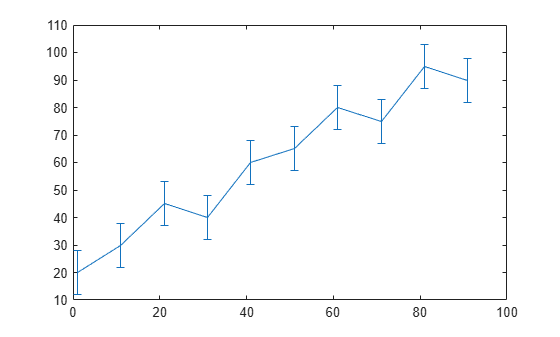Create a line plot with error bars at each data point. Vary the lengths of the error bars.

```x = 1:10:100; y = [20 30 45 40 60 65 80 75 95 90]; err = [5 8 2 9 3 3 8 3 9 3]; errorbar(x,y,err)```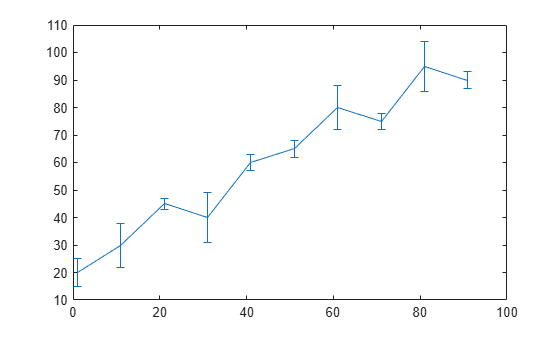Create a line plot with horizontal error bars at each data point.

```x = 1:10:100; y = [20 30 45 40 60 65 80 75 95 90]; err = [1 3 5 3 5 3 6 4 3 3]; errorbar(x,y,err,'horizontal')```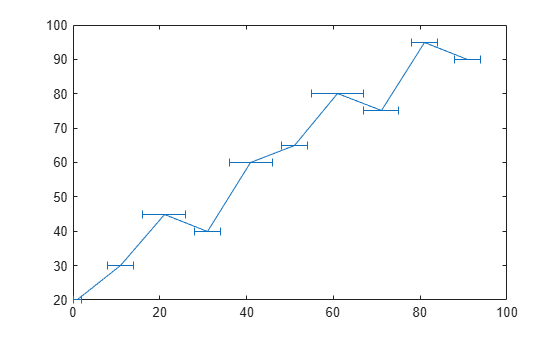Create a line plot with both vertical and horizontal error bars at each data point.

```x = 1:10:100; y = [20 30 45 40 60 65 80 75 95 90]; err = [4 3 5 3 5 3 6 4 3 3]; errorbar(x,y,err,'both')```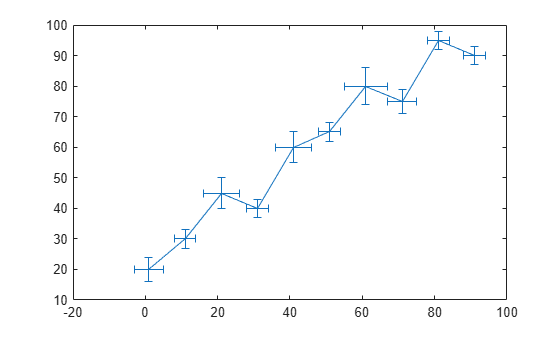Plot vectors `y` versus `x`. At each data point, display a circle marker with both vertical and horizontal error bars. Do not display the line that connects the data points by omitting the line style option for the `linespec` input argument.

```x = 1:10:100; y = [20 30 45 40 60 65 80 75 95 90]; err = [4 3 5 3 5 3 6 4 3 3]; errorbar(x,y,err,'both','o')```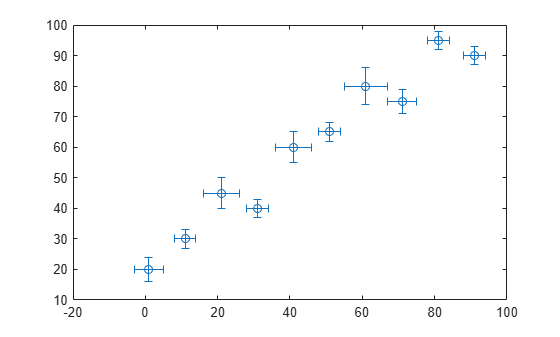Display both vertical and horizontal error bars at each data point. Control the lower and upper lengths of the vertical error bars using the `yneg` and `ypos` input argument options, respectively. Control the left and right lengths of the horizontal error bars using the `xneg` and `xpos` input argument options, respectively.

```x = 1:10:100; y = [20 30 45 40 60 65 80 75 95 90]; yneg = [1 3 5 3 5 3 6 4 3 3]; ypos = [2 5 3 5 2 5 2 2 5 5]; xneg = [1 3 5 3 5 3 6 4 3 3]; xpos = [2 5 3 5 2 5 2 2 5 5]; errorbar(x,y,yneg,ypos,xneg,xpos,'o')```Create a plot of datetime values with error bars in duration units.

```x = 1:13; y = datetime(2018,5,1,1:13,0,0); err = hours(rand(13,1)); errorbar(x,y,err)```Create a line plot with error bars. At each data point, display a marker. Control the appearance of the marker using name-value pair arguments. Use `MarkerSize` to specify the marker size in points. Use `MarkerEdgeColor` and `MarkerFaceColor` to specify the marker outline and interior colors, respectively. Set the colors to either a character vector of a color name, such as `'red'`, or an RGB triplet.

```x = linspace(0,10,15); y = sin(x/2); err = 0.3*ones(size(y)); errorbar(x,y,err,'-s','MarkerSize',10,... 'MarkerEdgeColor','red','MarkerFaceColor','red')```Control the size of the caps at the end of each error bar by setting the `CapSize` property to a positive value in points.

```x = linspace(0,2,15); y = exp(x); err = 0.3*ones(size(y)); errorbar(x,y,err,'CapSize',18)```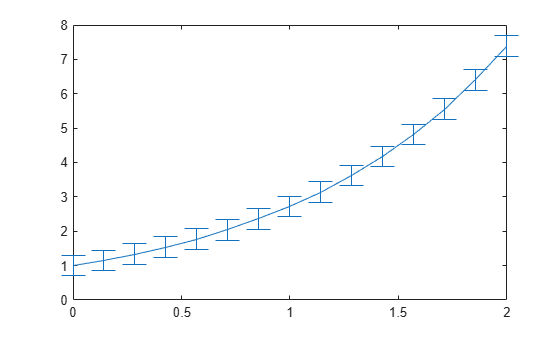Create a line plot with error bars. Assign the errorbar object to the variable `e`.

```x = linspace(0,10,10); y = sin(x/2); err = 0.3*ones(size(y)); e = errorbar(x,y,err)```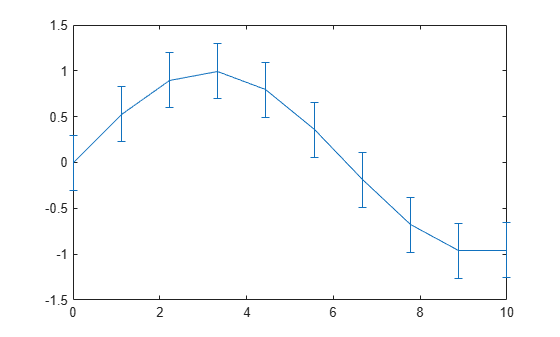```e = ErrorBar with properties: Color: [0 0.4470 0.7410] LineStyle: '-' LineWidth: 0.5000 Marker: 'none' XData: [0 1.1111 2.2222 3.3333 4.4444 5.5556 6.6667 7.7778 ... ] YData: [0 0.5274 0.8962 0.9954 0.7952 0.3558 -0.1906 ... ] XNegativeDelta: [1x0 double] XPositiveDelta: [1x0 double] YNegativeDelta: [0.3000 0.3000 0.3000 0.3000 0.3000 0.3000 0.3000 ... ] YPositiveDelta: [0.3000 0.3000 0.3000 0.3000 0.3000 0.3000 0.3000 ... ] Show all properties ```

Use `e` to access properties of the errorbar object after it is created.

```e.Marker = '*'; e.MarkerSize = 10; e.Color = 'red'; e.CapSize = 15;```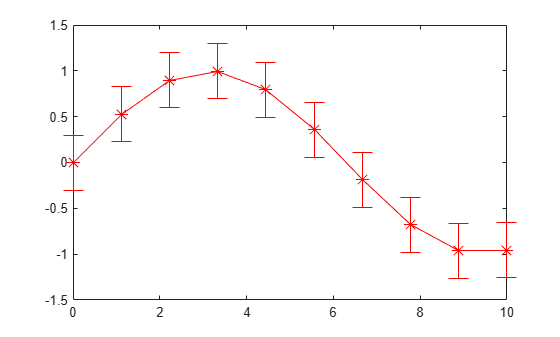## Input Arguments

collapse all

y values, specified as a vector or a matrix.

• If `y` is a vector, then `errorbar` plots one line.

• If `y` is a matrix, then `errorbar` plots a separate line for each column in `y`.

Example: `y = [4 3 5 2 2 4];`

Data Types: `single` | `double` | `int8` | `int16` | `int32` | `int64` | `uint8` | `uint16` | `uint32` | `uint64` | `logical` | `categorical` | `datetime` | `duration`

x values, specified as a vector or a matrix. `x` must be the same size as `y`.

Example: `x = 0:10:100;`

Data Types: `single` | `double` | `int8` | `int16` | `int32` | `int64` | `uint8` | `uint16` | `uint32` | `uint64` | `logical` | `categorical` | `datetime` | `duration`

Error bar lengths for symmetrical error bars, specified as a vector or a matrix. `err` must be the same size as `y`. If you do not want to draw an error bar at a particular data point, then specify the length as `NaN`.

The data type of the error bar lengths must be compatible with the corresponding plot data. For example, if you plot `datetime` values, the error bars for those values must be `duration` values.

Data Types: `single` | `double` | `int8` | `int16` | `int32` | `int64` | `uint8` | `uint16` | `uint32` | `uint64` | `logical` | `duration`

Error bar lengths in the negative direction, specified as a vector or matrix the same size as `y` or as an empty array `[]`.

• For vertical error bars, `neg` sets the length of the error bars below the data points.

• For horizontal error bars, `neg` sets the length of the error bars to the left of the data points.

If you do not want to draw the lower part of the error bar at a particular data point, then specify the length as `NaN`. If you do not want to draw the lower part of the error bar at any data point, then set `neg` to an empty array.

The data type of the error bar lengths must be compatible with the corresponding plot data. For example, if you plot `datetime` values, the error bars for those values must be `duration` values.

Data Types: `single` | `double` | `int8` | `int16` | `int32` | `int64` | `uint8` | `uint16` | `uint32` | `uint64` | `logical` | `duration`

Error bar lengths in the positive direction, specified as a vector or matrix the same size as `y` or as an empty array `[]`.

• For vertical error bars, `pos` sets the length of the error bars above the data points.

• For horizontal error bars, `pos` sets the length of the error bars to the right of the data points.

If you do not want to draw the upper part of the error bar at a particular data point, then specify the length as `NaN`. If you do not want to draw the upper part of the error bar at any data point, then set `pos` to an empty array.

The data type of the error bar lengths must be compatible with the corresponding plot data. For example, if you plot `datetime` values, the error bars for those values must be `duration` values.

Data Types: `single` | `double` | `int8` | `int16` | `int32` | `int64` | `uint8` | `uint16` | `uint32` | `uint64` | `logical` | `duration`

Vertical error bar lengths below the data points, specified as a vector or matrix the same size as `y` or as an empty array `[]`.

If you do not want to draw the lower part of the error bar at a particular data point, then specify the value as `NaN`. If you do not want to draw the lower part of the error bar at any data point, then set `yneg` to an empty array.

The data type of the error bar lengths must be compatible with the corresponding plot data. For example, if you plot `datetime` values, the error bars for those values must be `duration` values.

Data Types: `single` | `double` | `int8` | `int16` | `int32` | `int64` | `uint8` | `uint16` | `uint32` | `uint64` | `logical` | `duration`

Vertical error bar lengths above the data points, specified as a vector or matrix the same size as `y` or as an empty array `[]`.

If you do not want to draw the upper part of the error bar at a particular data point, then specify the length as `NaN`. If you do not want to draw the upper part of the error bar at any data point, then set `ypos` to an empty array.

The data type of the error bar lengths must be compatible with the corresponding plot data. For example, if you plot `datetime` values, the error bars for those values must be `duration` values.

Data Types: `single` | `double` | `int8` | `int16` | `int32` | `int64` | `uint8` | `uint16` | `uint32` | `uint64` | `logical` | `duration`

Horizontal error bar lengths to the left of the data points, specified as a vector or matrix the same size as `y` or as an empty array `[]`.

If you do not want to draw the left part of the error bar at a particular data point, then specify the length as `NaN`. If you do not want to draw the left part of the error bar at any data point, then set `xneg` to an empty array.

The data type of the error bar lengths must be compatible with the corresponding plot data. For example, if you plot `datetime` values, the error bars for those values must be `duration` values.

Data Types: `single` | `double` | `int8` | `int16` | `int32` | `int64` | `uint8` | `uint16` | `uint32` | `uint64` | `logical` | `duration`

Horizontal error bar lengths to the right of the data points, specified as a vector or matrix the same size as `y` or as an empty array `[]`.

If you do not want to draw the right part of the error bar at a particular data point, then specify the length as `NaN`. If you do not want to draw the right part of the error bar at any data point, then set `xpos` to an empty array.

The data type of the error bar lengths must be compatible with the corresponding plot data. For example, if you plot `datetime` values, the error bars for those values must be `duration` values.

Data Types: `single` | `double` | `int8` | `int16` | `int32` | `int64` | `uint8` | `uint16` | `uint32` | `uint64` | `logical` | `duration`

Error bar orientation, specified as one of these values:

• `'vertical'` — Vertical error bars

• `'horizontal'` — Horizontal error bars

• `'both'` — Vertical and horizontal error bars

Example: `errorbar(x,y,err,'horizontal')`

Line style, marker, and color, specified as a character vector or string containing symbols. The symbols can appear in any order. You do not need to specify all three characteristics (line style, marker, and color). For example, if you omit the line style and specify the marker, then the plot shows only the marker and no line.

Example: `'--or'` is a red dashed line with circle markers

Line StyleDescriptionResulting Line
`'-'`Solid line`'--'`Dashed line`':'`Dotted line`'-.'`Dash-dotted lineMarkerDescriptionResulting Marker
`'o'`Circle`'+'`Plus sign`'*'`Asterisk`'.'`Point`'x'`Cross`'_'`Horizontal line`'|'`Vertical line`'s'`Square`'d'`Diamond`'^'`Upward-pointing triangle`'v'`Downward-pointing triangle`'>'`Right-pointing triangle`'<'`Left-pointing triangle`'p'`Pentagram`'h'`HexagramColor NameShort NameRGB TripletAppearance
`'red'``'r'``[1 0 0]``'green'``'g'``[0 1 0]``'blue'``'b'``[0 0 1]``'cyan'` `'c'``[0 1 1]``'magenta'``'m'``[1 0 1]``'yellow'``'y'``[1 1 0]``'black'``'k'``[0 0 0]``'white'``'w'``[1 1 1]`Axes object. If you do not specify the axes, then `errorbar` plots into the current axes.

### Name-Value Arguments

Specify optional comma-separated pairs of `Name,Value` arguments. `Name` is the argument name and `Value` is the corresponding value. `Name` must appear inside quotes. You can specify several name and value pair arguments in any order as `Name1,Value1,...,NameN,ValueN`.

Example: `errorbar(y,err,'LineWidth',2)` specifies a line width of 2 points.

The properties listed here are only a subset. For a complete list, see ErrorBar Properties.

Length of caps at end of error bars, specified as a positive value in points.

Example: `errorbar(x,y,err,'CapSize',10)`

Line width, specified as a positive value in points, where 1 point = 1/72 of an inch. If the line has markers, then the line width also affects the marker edges.

The line width cannot be thinner than the width of a pixel. If you set the line width to a value that is less than the width of a pixel on your system, the line displays as one pixel wide.

## Extended Capabilities

### Properties

Introduced before R2006a